Juice box

In the box is 0.3 liters of juice. How many liters of juice contains 3 these boxes?

Result

V =  0.9 l

Solution:Leave us a comment of example and its solution (i.e. if it is still somewhat unclear...):Be the first to comment!To solve this example are needed these knowledge from mathematics:

Tip: Our volume units converter will help you with converion of volume units.

Next similar examples:

1. Milk bill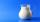Mrs Tara buys 2 liters of milk daily. If 1 liter of milk cost \$0.27. What will be her milk bill for 30 days.
2. If youIf you travel to work 22 days and it takes 29.2 minutes, how many minutes will it take to travel to work and back?
3. School trip6.A class went on a trip. Train ticket cost 6 euros, cable car ticket 6 euro. How many euros must pay classroom teacher for 23 students?
4. Seeds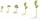Of the 72 wet seeds 7/8 germinated. How many seeds germinate?
5. Find the 6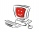Find the total cost of 10 computers at \$ 2100 each and 7 boxes of diskettes at \$12 each
6. Decimal expansion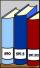Calculate: 2 . 1 + 0 . 10 + 7 . 10000 + 4 . 1000 + 6 . 100 + 0 . 100000 =
7. ProductResult of the product of the numbers 1, 2, 3, 1, 2, 0 is:
8. Expression 1What is 7+8-(5×2)+5-4+(6×(5-3)+6)-(8+10)-7+6?
9. ConcertOn a Concert were sold 150 tickets for CZK 360, 235 tickets for 240 CZK and 412 for 180 CZK. How much was the total revenues for tickets?
10. Conference 29 people attended a conference on behalf of their company. The conference fee was £520 per person and the company paid a total of £856 in travel costs for the 9 people. How much did the conference cost the company altogether?
11. NotebooksLiza a store owner buys 560 notebooks. He sold 3/8 of the notebook then she adds the stocks of notebook of 1/4 of the number of notebooks she bought. What is the total number of notebook she bought?
12. Operations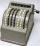Sum of the numbers 1.01 and 3.35 multiply by the difference of numbers 6.69 and 1.39.
13. Roman numerals +Add up the number writtens in Roman numerals. Write the results as a decimal number.
14. Roman numerals 2+Add up the number writtens in Roman numerals. Write the results as a roman numbers.Add this two mixed numbers: 1 5/6 + 2 2/11=At a weather centre, the temperature at midnight was -2 degree Celsius and by noon it had raised 4 degree Celsius. What is the new temperature?Added together and write as decimal number: LXVII + MLXIV